Science Innovation
Volume 3, Issue 6, December 2015, Pages: 92-99

Employment of the Limit-States Method and the Safety Theory in Geotechnical Design

Sc. Gilberto Quevedo Sotolongo*, Ana V. González – Cueto Vila, Luis O. Ibañez Mora

Faculty of Constructions, Central University of Las Villas, Carretera a Camajuaní, Km 5y½, Santa Clara, Villa Clara, Cuba(Sc. G. Q. Sotolongo)

Sc. Gilberto Quevedo Sotolongo, Ana V. González – Cueto Vila, Luis O. Ibañez Mora. Employment of the Limit-States Method and the Safety Theory in Geotechnical Design.Science Innovation.Vol.3, No. 6, 2015, pp. 92-99. doi: 10.11648/j.si.20150306.15

Abstract: In this paper, a methodology is proposed to apply the Safety Theory in the obtaining of safety factor systems required in the geotechnical design of the foundations using Limit-States Method. The entire mathematical formulation that facilitates the application of the safety theory as well as the integral methodology for the safety factor systems to be used is defined. In addition, a practical example is developed where it is allowed to value the security introduced in the design.

Keywords: Limit-States Method, Safety Factors, Foundation, Safety Theory

1. Introduction

The application of the limit-state method in geotechnical design is current research issue. Geotechnical design using the LSM has been mainly developed by more advanced countries in this field, such as Russia, Denmark, United States and Canada (Becker,1996), (Meyerhof,1970 and 1995). In Cuba, the study of the LSM started out in the mid eighties, and continues up to the present. Its main objective is to establish general methodology for geotechnical design using the limit-state method in the Cuban conditions. The paper present the main results obtained in this field of research in Cuba, like the introduction that has been done on the limit state method in engineering practice.

A general approach for the application of the limit-state method in the Cuban conditions is presented, bringing forward the entire mathematical procedure that supports the limit state method as well as its application to the main problems related to geotechnical design. The safety factor systems used in these designs are determined with the application of the probabilistic methods, safety theory, which are considered worldwide to be the most updated and rigorous from a scientific point of view. The entire mathematical formulation that facilitates the application of the safety theory as well as the integral methodology for the safety factor systems to be used is defined.

Finally, some of the main applications of there results during the last few years are shown. Thus, the economic and technical advantages of the proposed procedures are clearly demonstrated through the quantification of savings.

2. The General Methodology for the Application of the Limit State Method in Geotechnical Designing in Cuba

The general mathematical formulation for the introduction of the limit state method in geotechnical design in Cuba will be defined, which once later allow to establish, with a unique approach, the particular formulations for each one of the different geotechnical designs that are analyzed. As it is known, in the application of the limit-state method, the following limit states designs are defined:

1st limit state - The resistance limit state or stability limit state, known as the ultimate limit state

2nd limit state - The service limit state, which in the case of geotechnical design is converted into the deformation limit state

The design equation for the 1st limit state which will be employed is that which uses a system of three factors of partial safety, such as shown in formula (1).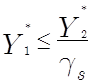(1)

where: Y1* - operating loads function, with their calculation values.

Y2*- bearing loads function, determined with the calculated values of the physical – mechanical soil properties.

Procedure for the application of the factor of safety system

Once the general equation to be used for the application of the limit state method in geotechnical design is defined, the procedure for the introduction of the partial safety factors for the determination of the Y1* y Y2*function must be established.

In the case of the operating loads, in the 1st limit state summarized in Figure 1, the calculation values of the loads are determined according to: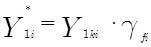(2)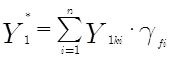(3)

where: Y1*i - calculation value of load i.

Y1k*i - characteristic value of load i.

Y1 i - mean value of load i.

gfi - safety factor of load i.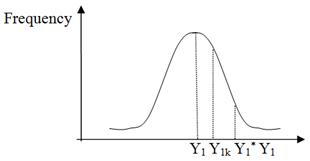Figure 1. Relationship between the functions Y1, Y1k and Y1*.

From figure 1 the expression that is used to determine the partial safety factor of the operating loads can be obtained, according to: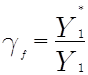(4)Figure 2. Relationship between the functions Y2* y Y2 for the1st limit state.

The proposed procedure for the determination of the bearing loads function with its calculation values Y2* differs from that which is used traditionally in structural design and establishes the direct application of the safety factors of the physical-mechanical soil properties to its mean values, as shown in figure 2.

From Figure 2 it is deduced that the partial factor of the bearing loads gg is obtained from the relationship between the evaluated bearing capacity function for the mean values of the physical-mechanical soil properties and for its calculation values.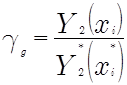(5)

Relationship between the partial factors of the limit state method and the global safety factor of the global safety factor method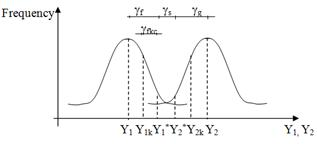Figure 3. Relationship between the functions Y1, Y1k, Y1*, Y2*, Y2k and Y2 and the partial factors.

For the calibration of the safety factor system of to be used by the limit state method, it is necessary to establish procedures for determining the global safety factor K that is introduced in the limit state method with the use of partial factors. The relationship between these partial and global factors can be observed in figure 3 and can be determined using equation (6).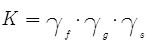(6)

In order to carry out the comparison between the global factors introduced by the limit state method and the global safety factor method, it is convenient to define the value of the global safety factor, measured from the characteristic values of the operating loads to the mean values of the bearing loads, Kkm, which is determined according to: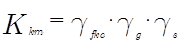(7)

3. Formulation of the Limit State Method in Diverse Geotechnical Designs in Cuba

Taking as a basis the general methodologies previously defined, for the application of the limit state method and the safety theory in geotechnical design, diverse formulations of specific designs have been done (Alvarez, 1998), (González-Cueto 1997 y 2000), (Ibañez, 2001), (Oliva, 1999), (Quevedo,1987, 1988, and 1989), such as foundations, retaining walls, slopes and piles as a result of these investigations we rely on methodologies established with a unique approach. This fact has made it possible to place Cuba among the countries with more development in this field.

Foundations.

1st Limit state: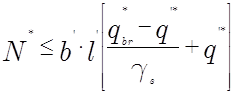(8)

Retaining walls.

Overturning:(9)

Sliding :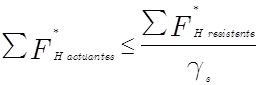(10)

Slopes.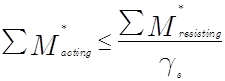(11)

Piles.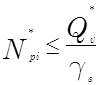(12)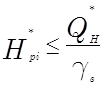(13)

4. General Methodology for the Application of the Safety Theory in Geotechnical Design in Cuba

From the different approaches analyzed for the application of the probabilistic methods, known by some authors as the safety theory, a design equation that defines the comparison between the levels of safety obtained in the design Hdesign and the level of safety required Hrequired would be considered as the starting point.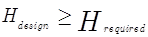(14)

General mathematical formulation for the application of the safety theory

Once the basic equation for the application of the safety theory has been defined, it is necessary to develop the completely mathematical foundation on which its practical use is based, its fundamental expressions being the following: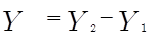(15)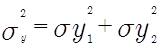(16)

Where: sY - deviation of the resulting function Y

sY1 - deviation of the function Y1

sY2 - deviation of the function Y2

It is possible to demonstrate that the level of safety is determined based on evaluating the integral of Laplace’s function fn between -b and +¥, as shown: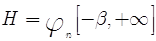(17)

By considering a normal distribution for the function Y and its symmetry properties, and knowing that the evaluated distribution between 0 and +¥ is equal to 0.5, it is possible to define the level of safety as: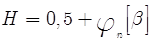(18)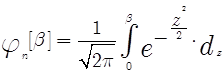(19)

By doing mathematical transformations with the previous equations and using the concept of additional safety factor k, the equation, which relates the level of safety H with the global safety factor k, can be obtained.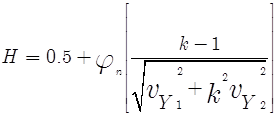(20)

To this end, equation (20) will be the basic equation for the application of the safety theory. Thus, once required security level value Hreq is defined, it is very easy to determine the optimum safety factor, which should be used in the design, see figure 4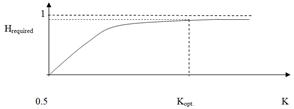Figure 4. Relationship between the safety level H and the global safety factor K.

In order to be able to use equation (20), it is necessary to establish the procedure to obtain the factors for variation of the functions Y1 and Y2, which in turn consist of various random variables.

5. Application of the Safety Theory in the Calibration of the Safety Factors System to be Employed in the Design of Foundations

In order to show the results obtained in the application of the safety theory in geotechnical design in Cuba, the procedure followed in the case of foundations in soils that are predominantly cohesive will be displayed in detail. This is the first research work on the subject that is done in the country (Quevedo, 1987) and it is the basis for further generalization for the solution of similar geotechnical problems.

For the application of the safety theory, equation (8) has been simplified, considering that the load eccentricity exists in only one plane of symmetry, therefore l’= l, and that the additional safety factor gs affects the gross load capacity qbr*. Based on this, the following design equation is obtained: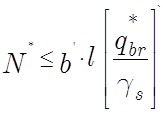(21)

Next, the general methodology developed for the application of the safety theory will be described.

I). Definition of the general parameters to be considered in the analysis

I.1). Statistical characterization of all the random parameters considered

In the case of geotechnical design the random parameters considered are operating loads and physical–mechanical soil properties, coefficients of variation of the internal friction angle vtgj , of the cohesion vc, of the specific weight vg, of the dead load vcm, of the live load vcv and of the extreme wind load vwind.

I.2) Design of the theoretical experiment

The variables analyzed for the application of the safety theory are cohesion c, internal friction angle j, specific weight g, load eccentricity e, inclination of the load d and the depth of the foundation d.

I.3) Definition of the required safety level

In the case of geotechnical design via the 1st limit state, the Hrequired to be used in the application of the probabilistic methods is:

Hrequired = 0.98.

II) Determination of the global safety design factor Kdesign and the optimum safety factor Koptimum.

II.1) Development of the mathematical apparatus

The expressions to determine the partial factors of operating loads gf and of bearing loads gg introduced in the design are: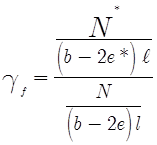(22)(23)

In this way all the expressions used to determine de gf y gg, are defined, whilst the global safety design factor Kdesign is obtained from the following: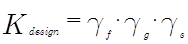(24)

In order to determine the factor of optimum safety that must be introduced in the design Koptimum, it is necessary to initially define the functions Y1 and Y2.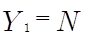(25)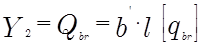(26)

Thus, obtaining the equation that relates the level of safety H to the factor of global safety K, in the case of the design of foundations using the load capacity is defined by: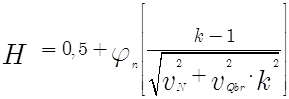(27)

The coefficients of variation of the vertical operating load vN and the load capacity vQbr are determined accordingly: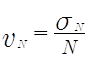(28)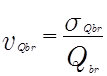(29)

where: sN - deviation of the vertical operating load.

sQbr - deviation of the load capacity in the base of the foundation

In order to determine sQbr , Qbr the method of linearization of the function and the general theorem of deviation , known by as the method of development in Taylor’s series  is applied to the expression for Qbr, thus obtaining: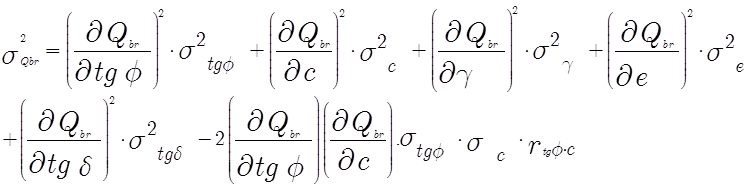(30)

Finally, the deviation of the vertical loads is determined from: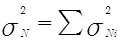(31)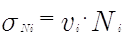(32)

II.2) Creation of a computational basis

II.3) Obtainment of the values Kdesign and Koptimum for the defined combinations

Once the analysis of all the defined variants is done, with the help of the computational basis created, it is necessary to consolidate the substantial information obtained in a way that facilitates its assessment and the arrival to partial conclusions of this part of the research.

In the case of the problem under study, the results obtained were summarized in Table 1.

Table 1. Values of the factors gf, gg, Kdesign y Koptimum.

 Variation coefficients Variation interval gf gg Kdesign Koptimum vtgj = 0.07  vc = 0.138 1.48 ~ 1.76 1.28 ~1.51 1.89 ~ 2.65 1.3 ~ 1.6 vtgj = 0.20  vc = 0.26 1.42 ~ 1.68 1.65 ~ 2.61 2.34 ~ 4.38 1.5 ~ 2.1 vtgj = 0.26  vc = 0.336 1.37 ~ 1.63 1.99 ~ 3.78 2.72 ~ 6.16 1.9 ~ 2.6

II.4) Assessment of the results and formulation of partial conclusions

The results obtained demonstrate that in these designs the values of the factors of global safety introduced, Kdesign, are always greater than the optimum, Koptimum, necessary for the achievement of the required level of safety Hrequired, which indicates that, using the proposed procedure for the application of the partial factors in the limit state method, factors of safety greater than those required are obtained and therefore the designs are irrational.

III) Calibration of the factors of safety

III.1) Analysis of the influence of the main variables in the values of Kdesign and Koptimum

In this research, the analysis of the influence of all the random variables considered in the values of Kdesign and Koptimum is done.

In figure 5 the results from the analysis for the influence of the coefficient of variation vtgj is shown, but integrated in that same figure is the variation of Kdesign and Koptimum for all the combinations analyzed, which allows the comparative assessment of the laws of variation of both parameters in relation to the variable of study.

Furthermore an assessment of the influence of the rest of the variables, the variation coefficient of cohesion vc, the eccentricity e and the angle of inclination of the load d, on the values of Kdesign and Koptimum was done.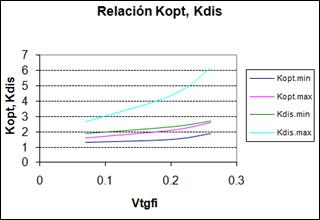Figure 5. Relationship of Koptimum and the Kdesign with vtgfi.

III.2) Determination of the worst combinations with regard to the relationship Kdesign and Koptimum

The worst combinations obtained are:

Internal friction angle The least possible j = 5º

Load eccentricity The largest possible e = b/3

Load inclination The largest possible d = 10º

III.3) Calibration of the safety factor system to be used in the limit state method

This is a genuine engineering process, and by using all the available information from the previous analyses a new safety factor system is established, which will be used for the design of foundations via the limit state method, in such a way that it is guaranteed for all the possible cases of safety required, obtaining for those most critical the fulfillment of Kdesign = Koptimum and therefore Hdesign = Hrequired.

Table #2. Maximum values of gtgj and ggc for cohesive soils in the 1stlimit state.

 Variation coefficient Maximum values of gtgj or ggc vtgj £ 0.20 gtgjmax = 1.20 vc £ 0.26 ggcmax = 1.40 vtgj > 0.20 gtgjmax = 1.25 Vc > 0.26 ggcmax = 1.45

For foundation design in cohesive soils, via bearing capacity and applying the limit state method, the results from the calibration is summarized in table # 2.

III.4). Obtainment of new values of Kdesign with the calibrated factors

With the new safety factor system that has been proposed, all the values of Kdesign are determined.

Table #3. Variation of the coefficients gf, gg, Kdesign y Koptimum obtained.

 Variation coefficient. Variation interval gf gg Kdesign Koptimum vtgj £ 0.20 1.42 ~ 1.76 1.21 ~ 1.92 1.71 ~ 3.38 1.3 ~ 2.1 vc £ 0.26 vtgj > 0.20 1.37 ~ 1.63 1.44 ~ 2.17 1.98 ~ 3.54 1.9 ~ 2.6 vc > 0.26

An analysis similar to that which was carried out in III.1 was done with the new factors of safety, thus figure 6 shows how these factors vary with respect to those obtained previously without the limitation of the coefficients.

III.5) Assessment of the results and formulation of conclusions

If we analyze comparatively figures 5 and 6, it is possible to see that once the values of factors gtgj and ggc are limited, the Kdesign reduces, mainly maximum values, while the Koptimum hardly changes, thus obtaining a zone between the Koptimummax and Kdesignmin curves, where the critical combinations for which the factors have been calibrated are sure to be located.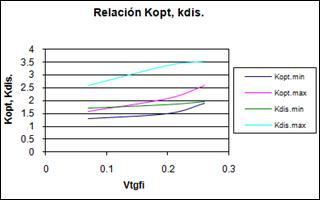Figure 6. Relationship between Koptimum and Kdesign with vtgfi.

IV). Practical validation of the results.

For the practical assessment of the main contributions made to the design methodology of foundations in cohesive soils, the results obtained in the foundation design of an industrial factory from a typical project will be analyzed.

The foundation designs were done using three procedures, the limit state method following to the proposed methodology, with the safety factor system obtained from the application of the probabilistic methods, which will be identified as the proposed limit state method; the limit state method with the factor of safety system obtained from the application of statistics, which will be identified as the limit state method without limiting, and the global safety factor method with Kkm= 3, which will be identified as the global safety factor method. The results obtained from these designs are shown in table # 4.

Table 4. The design results.

 Foundation I. Proposed LSM II. Previous LSM % savings I ~ II III. GSFM % savings I ~ III B(m) Kmm Kkm B(m) Kmm Kkm B(m) Kmm Kkm Internal 2.30 2.25 1.80 2.40 2.41 1.93 8.7 % 2.90 3.75 3.0 37.9 % External 2.60 2.76 2.22 2.65 2.80 2.24 5.0 % 2.90 3.75 3.0 23.0 %

Table # 5. General safety factors system for cohesive soils.

 Cohesive soils. ( c ¹ 0 ; j £ 25º ) Applications Limit state/ Level of safety Factors ggtgjmax or ggcmax Vc£ 0.26 vc> 0.26 vtgj£ 0.20 Vvgj> 0.20 Foundations.Slopes. 1stLimit state/H=0.98 1stLimit state/H=0.95 ggc=1.40 ggc=1.45 ggtgj= 1.20 ggtgj= 1.25 Foundations Retaining walls. 2ndLimit state/H=0.85 1stLimit state/H=0.98 ggc=1.30 ggc=1.35 ggtgj= 1.10 ggtgj= 1.15 Piles. 1stLimit state/H=0.98 ggc=1.20 ggc=1.40 - -

From the results obtained a number of conclusions that confirm several of the hypotheses considered in the present task can be drawn, the main ones being the following:

In interior foundations, which work at axial loading with eccentricity zero, using the proposed limit state method the factors of safety are required to be lower than those for the exterior foundations which have eccentric loads.

The rationality of the proposed limit state method in relation to the global safety factor method which has been used frequently the engineering practice is demonstrated, obtaining savings in terms of direct cost of the foundation ranging from 23 ~ 38%, depending on the type of foundation and the type of soil.

Safety Factors System for geotechnical design using the limit state method in Cuba

Following the methodology developed for the application of the probabilistic methods with the objective of obtaining the safety factors system to be used in geotechnical design using the limit state method, a group of research work have been carried out in Cuba, (Alvarez, 1998), (González-Cueto 1997 y 2000), (Ibañez, 2001), (Oliva, 1999), (Quevedo, 1987, 1988, 1989 and 2000), which included the study of the following problems:

Foundations

Retaining walls

Slopes

Piles

Table # 5 shows the unified safety factors system, for cohesive soils, likewise a table was also prepared for frictional soils to be used in geotechnical design via the limit state method.

6. Conclusions

1.   Based on the international experience and the results from research, a proposed of a general procedure for the introduction of the limit state method in geotechnical design in Cuba is made. This proposal is characterized by the use of a system of three factors of partial safety, one related to the acting loads, the other to the physical–mechanical soil characteristics and the third to additional safety, from which the general mathematical apparatus which supports its rational application in any geotechnical design problem is defined.

2.   The general mathematical apparatus is established for the application of the safety theory and probabilistic methods, thus proposing a solution for various problems that are not addressed in the international literature, such as the analysis of functions with correlated random variables. For the first time the coincidence of approaches followed by the two main schools the western and the Russian, on the subject is demonstrated when it comes to applying probabilistic methods. The mathematical apparatus created can be used for any geotechnical problem.

3.   A general methodology is proposed for the application of the probabilistic methods in geotechnical design, which is characterized by the creative combination of the mathematical precision of this method with the engineering solutions, in such a way that the results obtained can be introduced easily in practice.

4.   Based on this research and on the assessment of the international experience, it is possible to summarize the statistic characterization of the main random variables that take part in geotechnical design, the operating loads and the physical-mechanical soil properties, which facilitate the application of the probabilistic methods in any geotechnical problem to be tackled.

5.   Based on the results obtained from the application of the safety theory in the determination of the factor of safety system of various geotechnical problems, foundations, walls, slopes and piles, the practical effectiveness of the general proposed methodology is demonstrated, stating furthermore general factors of safety system, distinctive for cohesive and frictional soils, applicable to the geotechnical design of foundations using both limit states of any of the problems under study.

6.   Using the results obtained from the application of the general methodology for the geotechnical design of foundations, its technical-economical effectiveness is demonstrated, the influence of each one of the new aspects introduced in the design thus quantified obtaining savings ranging from 15 ~ 40 % for direct cost of the foundation, depending on the procedure used in the design with which it is compared and the peculiarities of the soils and the operating loads of the structure under study.

References

1. Álvarez Gil, L. "La estabilidad de cortinas de presas de tierra mediante la solución de los estados tenso-deformacionales y la aplicación de la teoría de seguridad" Dr. Gilberto Quevedo Sotolongo, tutor-TGC; UCLV-(Const.)127 pp, 1998.
2. Becker D. E. "Eighteenth Canadian Geotechnical Colloquium: Limit States Design for Foundations. Part I. An overview of the foundation design process." Canadian Geotechnical Journal 33: 956 – 983. 1996.
3. Blazquez Martínez, R. Geoestadística aplicada a la mecánica de suelos / R. Martínez Blazquez – CEDEX: Madrid, - 1949 pág. 1984.
4. González - Cueto, Ana Virginia. "Diseño de cimentaciones superficiales en arenas. Aplicación de la Teoría de Seguridad." Dr. Ing. Gilberto Quevedo Sotolongo, tutor--TM; UCLV--(Const). 70 pág. 1997.
5. González - Cueto, Ana Virginia. "Diseño geotécnico de cimentaciones superficiales en arenas."Dr. Ing. Gilberto Quevedo Sotolongo, tutor--TGC; UCLV--(Const). 132 pág. 2000.
6. Ibáñez, L.O.. "Análisisdel comportamiento geotécnico de las cimentaciones sobre pilotes sometidas a carga axial mediante la modelación matemática."Dr. Ing. Gilberto Quevedo Sotolongo, tutor--TGC; UCLV--(Const). 113 pág. 2001.
7. Meyerhof, G. G., "Safety Factors in Soil Mechanics" Canadian Geotechnical Journal. 7: 349 – 355, 1970.
8. Meyerhof, G. G., "Development of Geotechnical Limit State Design." Canadian Geotechnical Journal 32: 128 – 136, 1995.
9. Oliva González, A. "Análisis de la estabilidad y seguridad de taludes" Dr. Celestino González Niciesa, tutor, Dr. Gilberto Quevedo, consultante, -TGC, Universidad de Oviedo, 233pp, 1999.
10. Orr, T. L; Farrell, E. R. "Geotechnical Design to Eurocode 7" Springer-Verlag. London. 166 pag., 1999
11. Quevedo Sotolongo, G. "Aplicación de la Teoría de la Seguridad al diseño de las cimentaciones por deformación." Revista Ingeniería Estructural. 1(IX): 77 - 88; 1988.
12. Quevedo Sotolongo, G. "Aplicación de la Teoría de la Seguridad al diseño de las cimentaciones por estabilidad" Revista Ingeniería Estructural. 2(IX): 121 - 134; 1988.
13. Quevedo Sotolongo, G. "Aplicación del método de los estados límites en el diseño de las cimentaciones superficiales." Revista Ingeniería Estructural. 2(III): 95 - 106; 1987.
14. Quevedo Sotolongo, G. "Determinación del área de la base de cimentaciones superficiales: Método de cálculo."UCLV. (Const); 87 pág. 1989.
15. Quevedo Sotolongo, G. "Optimización y proyección de cimentaciones de edificaciones industriales en las condiciones de Cuba." – TGC; Moscú (MICI); -- 196 pág. 1987.
16. Quevedo Sotolongo G,; González-Cueto A. V. "Análisis de la seguridad en el diseño de cimentaciones en arenas. Criterio de estabilidad" Ingeniería Civil No. 119,CEDEX, Madrid, 2000.

 Contents 1. 2. 3. 4. 5. 6.
Article ToolsAbstractPDF(644K)